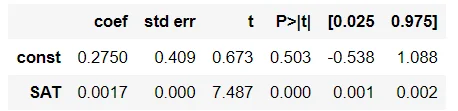07 Nov 2022

Posted on:

07 Nov 2022

5

# Resolved:Answer of the third question

I don't understand how we got the answer for question 3 in the regression quiz.

Instructor
Posted on:

07 Nov 2022

8

Hey Hassan,

Thank you for reaching out!

Let's examine the coefficients table from the OLS regression results:Using the value of the coefficients in front of const and SAT, let's write down the corresponding formula for linear regression, namely:
GPA = 0.2750 + 0.0017*SAT
We can see that the variable const has a p-value of 0.503 which makes it statistically insignificant. The question asks to make a prediction excluding such insignificant variables and that reduces the equation above down to
GPA = 0.0017*SAT
Now, plugging in SAT = 1850, we obtain the desired result.

Hope this helps!

Kind regards,
365 Hristina